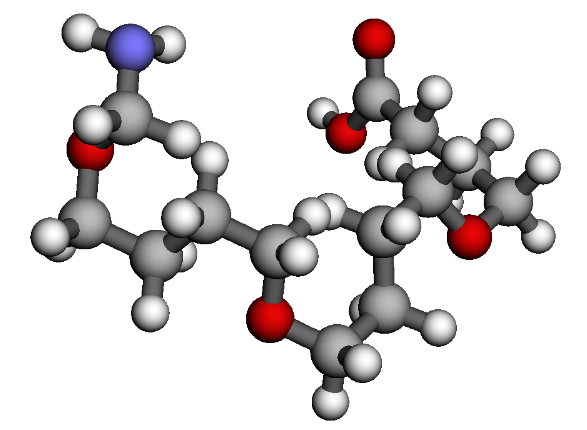# Polymer¶

Use two (2) different monomer units, A and B, to construct a polymer, capping it with a carboxylic acid and amine end group.

Import the required mbuild packages.

```import mbuild as mb
from mbuild.lib.recipes.polymer import Polymer
```

Create the monomer units comp_1 and comp_2 using SMILES strings. Set the chain as a `Polymer` class, adding comp_1 and comp_2 as the monomers A and B to the polymer.

Note

Setting the indices identifies which atoms will be removed and have ports created in their place.

```comp_1 = mb.load('CC', smiles=True) # mBuild compound of the monomer unit
comp_2 = mb.load('COC', smiles=True) # mBuild compound of the monomer unit
chain = Polymer()
indices=[2, -2],
separation=.15,
replace=True)

indices=[3, -1],
separation=.15,
replace=True)
```

Select the carboxylic acid and amine end groups that we want to use for the head and tail of the polymer. Then, build the polymer with three (3) iterations of the AB sequence, and the selected head and tail end groups.

```chain.add_end_groups(mb.load('C(=O)O',smiles=True),
index=3,
separation=0.15,
duplicate=False,